Скачать презентацию GLACIS DROP By CH VENKATARAMAIAH GLACIS DROP

• Количество слайдов: 9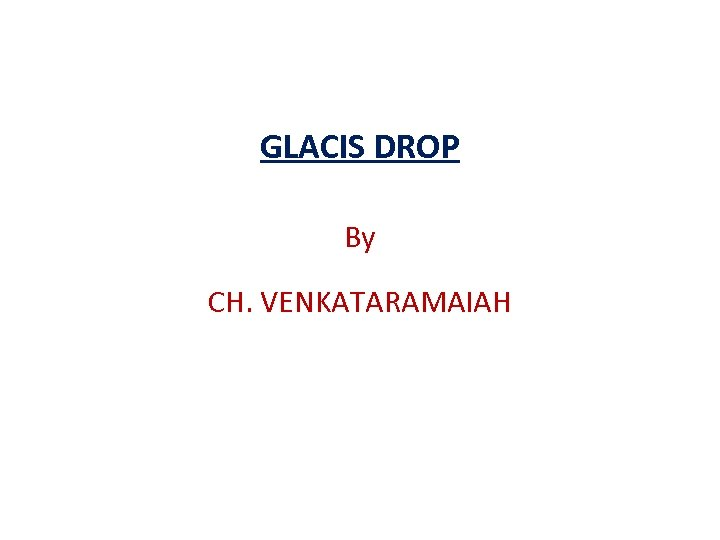GLACIS DROP By CH. VENKATARAMAIAH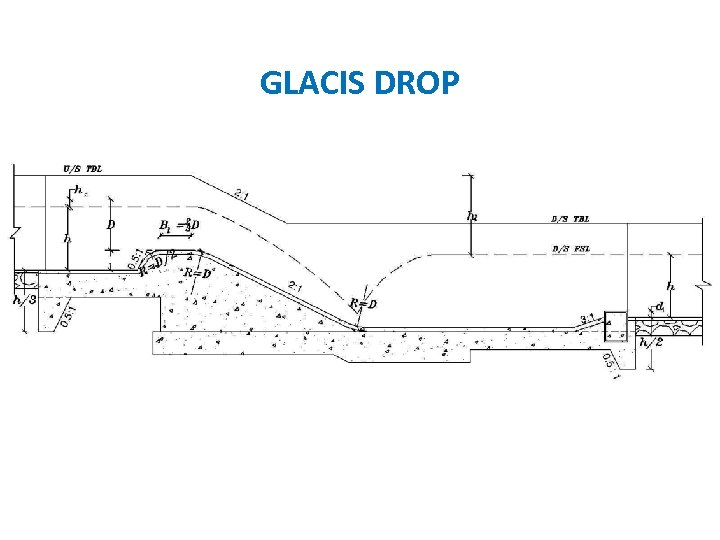GLACIS DROP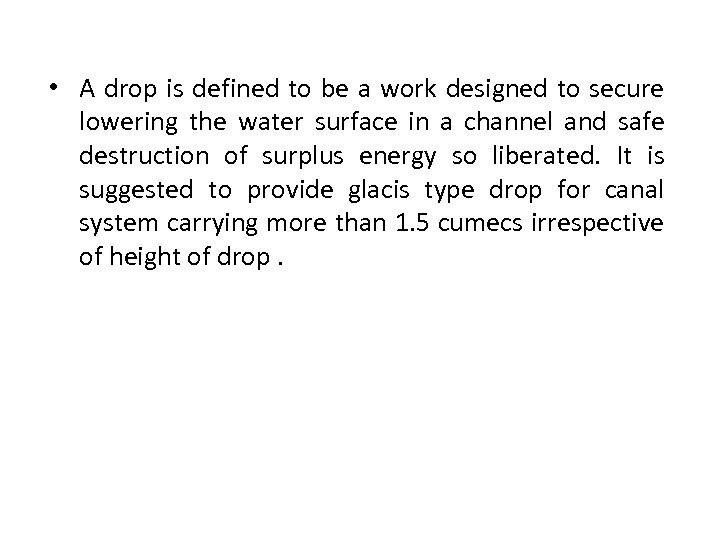• A drop is defined to be a work designed to secure lowering the water surface in a channel and safe destruction of surplus energy so liberated. It is suggested to provide glacis type drop for canal system carrying more than 1. 5 cumecs irrespective of height of drop.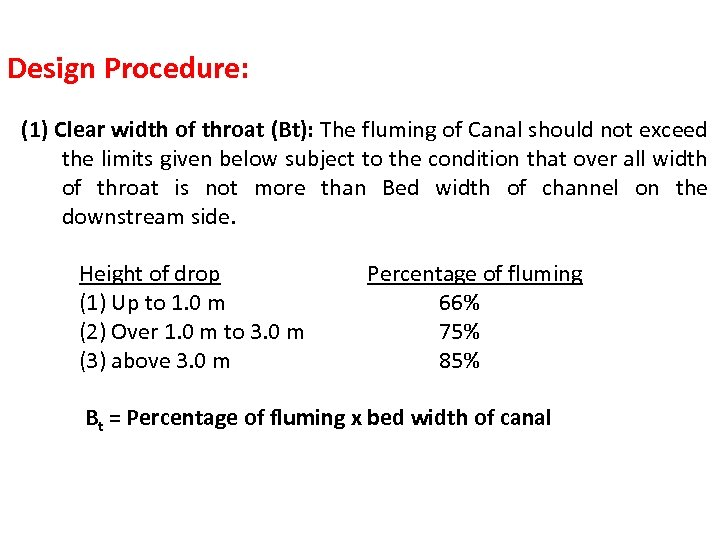Design Procedure: (1) Clear width of throat (Bt): The fluming of Canal should not exceed the limits given below subject to the condition that over all width of throat is not more than Bed width of channel on the downstream side. Height of drop (1) Up to 1. 0 m (2) Over 1. 0 m to 3. 0 m (3) above 3. 0 m Percentage of fluming 66% 75% 85% Bt = Percentage of fluming x bed width of canal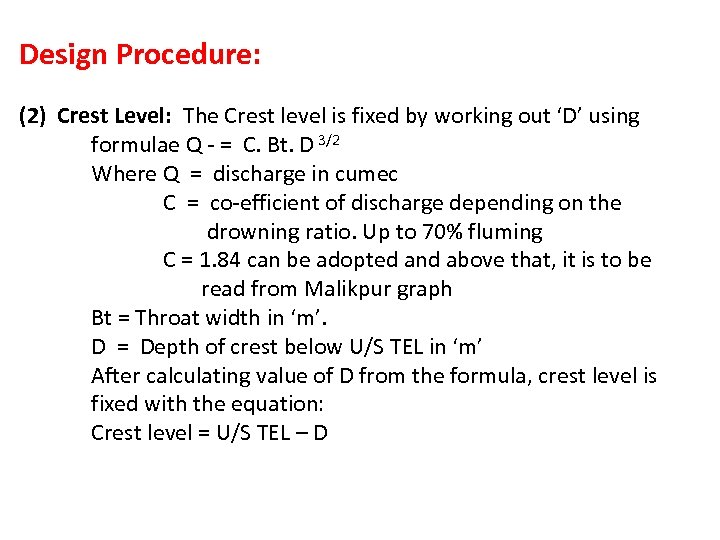Design Procedure: (2) Crest Level: The Crest level is fixed by working out ‘D’ using formulae Q - = C. Bt. D 3/2 Where Q = discharge in cumec C = co-efficient of discharge depending on the drowning ratio. Up to 70% fluming C = 1. 84 can be adopted and above that, it is to be read from Malikpur graph Bt = Throat width in ‘m’. D = Depth of crest below U/S TEL in ‘m’ After calculating value of D from the formula, crest level is fixed with the equation: Crest level = U/S TEL – D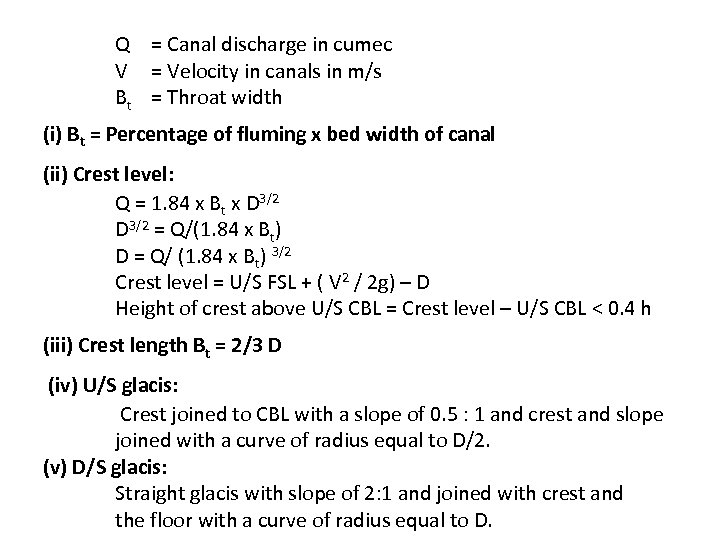Q = Canal discharge in cumec V = Velocity in canals in m/s Bt = Throat width (i) Bt = Percentage of fluming x bed width of canal (ii) Crest level: Q = 1. 84 x Bt x D 3/2 = Q/(1. 84 x Bt) D = Q/ (1. 84 x Bt) 3/2 Crest level = U/S FSL + ( V 2 / 2 g) – D Height of crest above U/S CBL = Crest level – U/S CBL < 0. 4 h (iii) Crest length Bt = 2/3 D (iv) U/S glacis: Crest joined to CBL with a slope of 0. 5 : 1 and crest and slope joined with a curve of radius equal to D/2. (v) D/S glacis: Straight glacis with slope of 2: 1 and joined with crest and the floor with a curve of radius equal to D.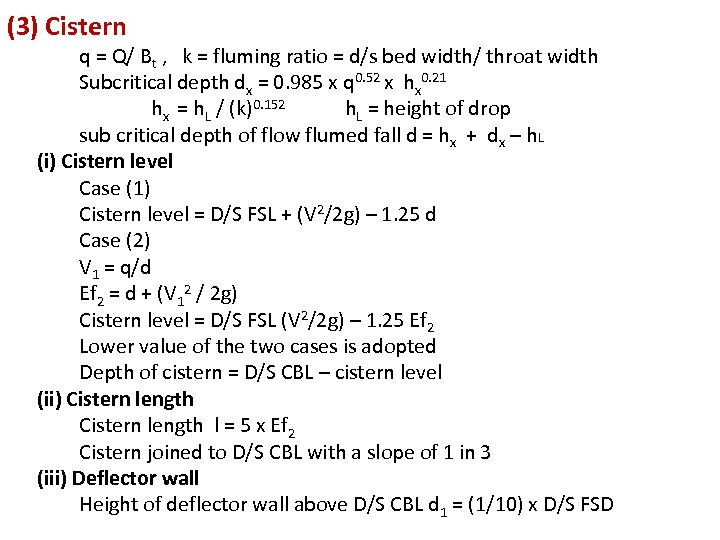(3) Cistern q = Q/ Bt , k = fluming ratio = d/s bed width/ throat width Subcritical depth dx = 0. 985 x q 0. 52 x hx 0. 21 hx = h. L / (k)0. 152 h. L = height of drop sub critical depth of flow flumed fall d = hx + dx – h. L (i) Cistern level Case (1) Cistern level = D/S FSL + (V 2/2 g) – 1. 25 d Case (2) V 1 = q/d Ef 2 = d + (V 12 / 2 g) Cistern level = D/S FSL (V 2/2 g) – 1. 25 Ef 2 Lower value of the two cases is adopted Depth of cistern = D/S CBL – cistern level (ii) Cistern length l = 5 x Ef 2 Cistern joined to D/S CBL with a slope of 1 in 3 (iii) Deflector wall Height of deflector wall above D/S CBL d 1 = (1/10) x D/S FSD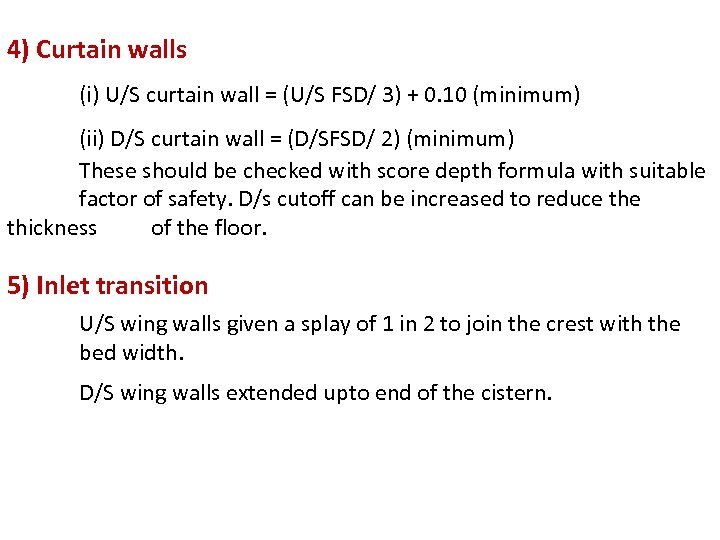4) Curtain walls (i) U/S curtain wall = (U/S FSD/ 3) + 0. 10 (minimum) (ii) D/S curtain wall = (D/SFSD/ 2) (minimum) These should be checked with score depth formula with suitable factor of safety. D/s cutoff can be increased to reduce thickness of the floor. 5) Inlet transition U/S wing walls given a splay of 1 in 2 to join the crest with the bed width. D/S wing walls extended upto end of the cistern.THANK YOU